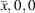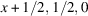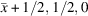International
Tables for
Crystallography
Volume E
Subperiodic groups
Edited by V. Kopský and D. B. Litvin

International Tables for Crystallography (2006). Vol. E, ch. 1.2, p. 16   | 1 | 2 |

## Section 1.2.11. Positions

V. Kopskýa and D. B. Litvinb*

aDepartment of Physics, University of the South Pacific, Suva, Fiji, and Institute of Physics, The Academy of Sciences of the Czech Republic, Na Slovance 2, PO Box 24, 180 40 Prague 8, Czech Republic, and bDepartment of Physics, Penn State Berks Campus, The Pennsylvania State University, PO Box 7009, Reading, PA 19610-6009, USA
Correspondence e-mail:  u3c@psu.edu

### 1.2.11. Positions

| top | pdf |

The entries under Positions (more explicitly called Wyckoff positions) consist of the General position (upper block) and the Special positions (blocks below). The columns in each block, from left to right, contain the following information for each Wyckoff position.

 (i) Multiplicity M of the Wyckoff position. This is the number of equivalent points per conventional cell. The multiplicity M of the general position is equal to the order of the point group of the subperiodic group, except in the case of centred layer groups when it is twice the order of the point group. The multiplicity M of a special position is equal to the order of the point group of the subperiodic group divided by the order of the site-symmetry group (see Section 1.2.12). (ii) Wyckoff letter. This letter is a coding scheme for the Wyckoff positions, starting with a at the bottom position and continuing upwards in alphabetical order. (iii) Site symmetry. This is explained in Section 1.2.12. (iv) Coordinates. The sequence of the coordinate triplets is based on the Generators. For the centred layer groups, the centring translations (0, 0, 0)+ and (1/2, 1/2, 0)+ are listed above the coordinate triplets. The symbol +' indicates that in order to obtain a complete Wyckoff position, the components of these centring translations have to be added to the listed coordinate triplets. (v) Reflection conditions. These are described in Section 1.2.13.

The two types of positions, general and special, are characterized as follows:

 (i) General position. A set of symmetrically equivalent points is said to be in a general position' if each of its points is left invariant only by the identity operation but by no other symmetry operation of the subperiodic group. (ii) Special position(s). A set of symmetrically equivalent points is said to be in a `special position' if each of its points is mapped onto itself by at least one additional operation in addition to the identity operation.

#### Example: Layer group c2/m11 (L18)

The general position 8f of this layer group contains eight equivalent points per cell each with site symmetry 1. The coordinate triplets of four points (1) to (4) are given explicitly, the coordinate triplets of the other four points are obtained by adding the components (1/2, 1/2, 0) of the c-centring translation to the coordinate triplets (1) to (4).

This layer group has five special positions with the Wyckoff letters a to e. The product of the multiplicity and the order of the site-symmetry group is the multiplicity of the general position. For position 4d, for example, the four equivalent points have the coordinates,,and. Since each point of position 4d is mapped onto itself by a twofold rotation, the multiplicity of the position is reduced from eight to four, whereas the order of the site symmetry is increased from one to two.